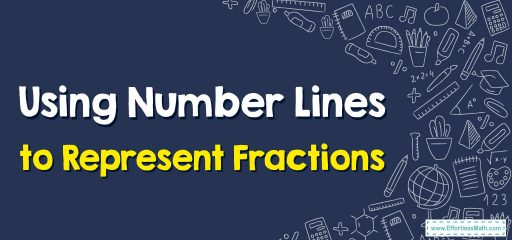# Using Number Lines to Represent FractionsA number line can be divided into several units to represent fractions.

Fractions are considered as a part of a whole and consist of two parts: numerator and denominator.

Numerator: It is a number on top.

Denominator: It is a number on the bottom.

## A step-by-step guide to representing fractions on the number lines

Step 1: First, divide the unit length between two numbers into some identical parts based on the denominator.

Step 2: Then count these identical parts and represent the numerator on the number line.

### Using Number Lines to Represent Fractions – Examples 1:

Graph $$fraction{2}{3}$$on the horizontal number line.

### Using Number Lines to Represent Fractions – Examples 2:

Graph $$fraction{5}{2}$$on the horizontal number line.

### What people say about "Using Number Lines to Represent Fractions - Effortless Math: We Help Students Learn to LOVE Mathematics"?

No one replied yet.

X
30% OFF

Limited time only!

Save Over 30%

SAVE $5 It was$16.99 now it is \$11.99